Daily Practice Problems
Class 9 Maths
Number SystemsQuestion 1:

Simplify: (√5 + √2)2

Question 2:

Identify a rational number among the following numbers:

2 + √2, 2√2, 0 and π

Question 3:

Express 23.43 in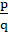form, where p, q are integers and q ≠ 0.

Question 4:

Simplify: √45 – 3√20 + 4√5

Question 5:

Rationalize the denominator of the following:

(i)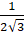(ii)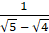Question 6:

If x +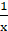= √3, find value of x3 +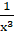.

Question 7:

Represent √17 on number line.

Question 8:

If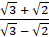= a + b√6, the find the value of a and b.

Question 9:

Represent √5 on number line.

Question 10:

Find out three rational numbers lying between 3 and 4.

Question 11:

If 72x + 3 = 1, then what is the value of x?

Question 12:

Convert 0.4777777.... inform.

Question 13:

Are the square roots of all positive integers irrational? If not, give an example of the square root of a number that is a rational number.

Question 14:

Visualize 3.765 on the number line, using successive magnification.

Question 15:

Simplify:        (i)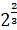*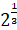(ii)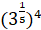(iii)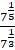Question 16:

What is the product of a rational and an irrational number?

(a) Always an integer

(b) Always a rational number

(c) Always an irrational number

(d) Sometimes rational and sometimes irrational

Question 17:

How many rational numbers and irrational numbers can be inserted between 4 and 5?

Question 18:

Write the following in decimal form and say what kind of decimal expansion each has:

(i)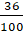(ii)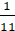(iii)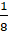Question 19:

Find the value of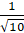when √10 = 3.162.

Question 20:

If x =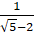, find the value of x2 – 4x – 1.

**********